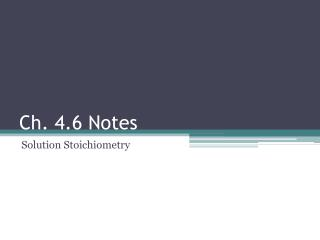DownloadDownload PresentationCh. 4.6 Notes

# Ch. 4.6 Notes

Download Presentation## Ch. 4.6 Notes

- - - - - - - - - - - - - - - - - - - - - - - - - - - E N D - - - - - - - - - - - - - - - - - - - - - - - - - - -
##### Presentation Transcript

1. Ch. 4.6 Notes Solution Stoichiometry

2. 4.6 • For solution stoichiometry, always want to work in moles, liters, molarity (mol/L) • (not grams, milliliters, etc.) • Remember to use mole ratio when moving between reactants and products

3. Titrations

4. 4.6 • Titrations • We know the molarity of a NaOH solution and want to know molarity of an HCl solution • KNOWN: molarity of NaOH, volume of HCl • UNKNOWN: molarity of HCl • How to find molarity of HCl: • Measure the volume of NaOH required to react completely with a known volume of HCl

5. 4.6 • Titrations (cont.) • What we found out: • Volume of NaOH already know molarity, can calculate moles • Next step: • Stoichiometry  calculate moles of HCl • HCl + NaOH  NaCl + H2O • Last step: • We have moles and volume of HCl, can calculate molarity (mol/L)

6. 4.6 • Practice! • An unknown solid acid is analyzed by titration. 1.75 g of the acid is weighed and dissolved in 150.0 mL of distilled water. The solution is titrated with 0.250 M KOH, and requires 19.07 mL of the KOH solution to reach the endpoint. What is the molar mass of the solid, assuming it is a strong acid and monoprotic?

7. 4.6 • Practice! • An unknown solid acid is analyzed by titration. 1.75 g of the acid is weighed and dissolved in 150.0 mL of distilled water. The solution is titrated with 0.250 M KOH, and requires 19.07 mL of the KOH solution to reach the endpoint. What is the molar mass of the solid, assuming it is a strong acid and monoprotic? • 0.250 M KOH = mol/.01907 L 0.00477 mol of KOH • 0.00477 mol of KOH would neutralize 0.00477 mol of our unknown acid • Molar mass = g/mol = 1.75 g/0.00477 mol = 367 g/mol